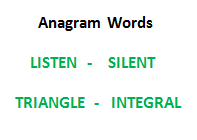# Check whether two Strings are Anagram of each other using HashMap in Java

Write a function to check whether two given strings are an Anagram of each other or not.

An anagram of a string is another string that contains the same characters, only the order of characters can be different.

For example, “abcd” and “dabc” are an Anagram of each other.## Recommended: Please try your approach on {IDE} first, before moving on to the solution.

Approach: Hashmaps can also be used to find if any two given strings are anagrams or not, by mapping the characters of each string to individual hashmaps and comparing them together.

Implementation:

 `// Java code to check whether two strings ` `// are Anagram or not using HashMap ` ` `  `import` `java.io.*; ` `import` `java.util.*; ` ` `  `class` `GFG { ` ` `  `    ``// Function to check whether two strings ` `    ``// are an anagram of each other ` `    ``static` `boolean` `areAnagram(String str1, String str2) ` `    ``{ ` ` `  `        ``HashMap hmap1 ` `            ``= ``new` `HashMap(); ` `        ``HashMap hmap2 ` `            ``= ``new` `HashMap(); ` ` `  `        ``char` `arr1[] = str1.toCharArray(); ` `        ``char` `arr2[] = str2.toCharArray(); ` ` `  `        ``// Mapping first string ` `        ``for` `(``int` `i = ``0``; i < arr1.length; i++) { ` ` `  `            ``if` `(hmap1.get(arr1[i]) == ``null``) { ` ` `  `                ``hmap1.put(arr1[i], ``1``); ` `            ``} ` `            ``else` `{ ` `                ``Integer c = (``int``)hmap1.get(arr1[i]); ` `                ``hmap1.put(arr1[i], ++c); ` `            ``} ` `        ``} ` ` `  `        ``// Mapping second String ` `        ``for` `(``int` `j = ``0``; j < arr2.length; j++) { ` ` `  `            ``if` `(hmap2.get(arr2[j]) == ``null``) ` `                ``hmap2.put(arr2[j], ``1``); ` `            ``else` `{ ` ` `  `                ``Integer d = (``int``)hmap2.get(arr2[j]); ` `                ``hmap2.put(arr2[j], ++d); ` `            ``} ` `        ``} ` ` `  `        ``if` `(hmap1.equals(hmap2)) ` `            ``return` `true``; ` `        ``else` `            ``return` `false``; ` `    ``} ` ` `  `    ``// Test function ` `    ``public` `static` `void` `test(String str1, String str2) ` `    ``{ ` ` `  `        ``System.out.println(``"Strings to be checked are:\n"` `                           ``+ str1 + ``"\n"` `+ str2 + ``"\n"``); ` ` `  `        ``// Find the result ` `        ``if` `(areAnagram(str1, str2)) ` `            ``System.out.println(``"The two strings are "` `                               ``+ ``"anagrams of each other\n"``); ` `        ``else` `            ``System.out.println(``"The two strings are not"` `                               ``+ ``" anagrams of each other\n"``); ` `    ``} ` ` `  `    ``// Driver program ` `    ``public` `static` `void` `main(String args[]) ` `    ``{ ` ` `  `        ``// Get the Strings ` `        ``String str1 = ``"geeksforgeeks"``; ` `        ``String str2 = ``"forgeeksgeeks"``; ` ` `  `        ``// Test the Strings ` `        ``test(str1, str2); ` ` `  `        ``// Get the Strings ` `        ``str1 = ``"geeksforgeeks"``; ` `        ``str2 = ``"geeks"``; ` ` `  `        ``// Test the Strings ` `        ``test(str1, str2); ` `    ``} ` `} `

Output:

```Strings to be checked are:
geeksforgeeks
forgeeksgeeks

The two strings are anagrams of each other

Strings to be checked are:
geeksforgeeks
geeks

The two strings are not anagram of each other
```

Related Article: Check whether two strings are anagram of each other

Attention reader! Don’t stop learning now. Get hold of all the important Java and Collections concepts with the Fundamentals of Java and Java Collections Course at a student-friendly price and become industry ready.

My Personal Notes arrow_drop_upCheck out this Author's contributed articles.

If you like GeeksforGeeks and would like to contribute, you can also write an article using contribute.geeksforgeeks.org or mail your article to contribute@geeksforgeeks.org. See your article appearing on the GeeksforGeeks main page and help other Geeks.

Please Improve this article if you find anything incorrect by clicking on the "Improve Article" button below.

Article Tags :
Practice Tags :

3

Please write to us at contribute@geeksforgeeks.org to report any issue with the above content.